# Electronic Polarization

Let us consider a single atom of atomic number Z. Say, +e coulomb is the charge of each proton in the nucleus and -e coulomb is the charge of each electron surrounds the nucleus. All orbiting electrons in the atom form a spherical cloud of negative charge surrounds the positively charged nucleus. The charge of nucleus is +Ze coulombs and charge of the negative cloud of electrons is -Ze coulombs. Let us also assume that the negative charge of the electrons cloud is homogeneously distributed on a sphere of radius R. When there is no influence of any external electric field, the center of this sphere and center of nucleus of the atom coincide. Now, say an external electric field of intensity E volt per meter is applied on the atom. Because of this external electric field the nucleus of the atom is shifted towards negative intensity of the field and the electron cloud is shifted towards the positive intensity of the field.

As due to influence of external electric field the center of nucleus and center of electrons cloud are separated, there will be an attraction force developed between them according to Coulomb’s law. Say, at the distance of separation of center of nucleus and electron cloud, x, the equilibrium is established. That means at distance of separation x, the forces acting on the nucleus or electron cloud due to external electric field and due to Coulomb law become same and opposite. It is obvious that the radius of nucleus is much much more than that of electron cloud. So in respect of electron cloud the nucleus can be considered as point charge. Hence, the electrostatic force acting on the nucleus would be +E.Z.e. Now the nucleus has been shifted from the center of electrons cloud by a distance x.

According to Gauss’s theorem, the force due to negative electron cloud acting upon the positive nucleus would only be due to the portion of the cloud enclosed by the sphere of radius x. The portion of the cloud outside the sphere of radius x does not apply any force on the nucleus. Now, the volume of the sphere of radius x is (4/3)πx3 and the volume of the sphere of radius R is (4/3)πR3.
Now total negative charge of the electron cloud is -Ze and we have already considered that it is uniformly distributed throughout the volume of the cloud.

Hence, the quantity of negative charge enclosed by the sphere of radius x is,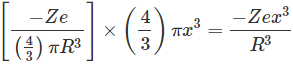Only this much charge will apply coulombic force on the nucleus. So, according to Coulomb’s law, the force would be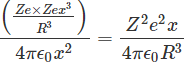At equilibrium condition,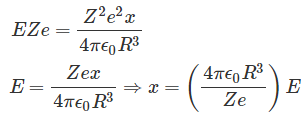Now the dipole moment of the nucleus is Zex as dipole moment is the product of charge of the nucleus and the distance of displacement. Now, putting the expression of x in the expression of dipole moment, we get,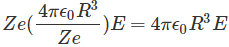The polarization is defined as the number of dipole moments per unit volume of the material. If N is the number of dipole moments per unit volume, the polarization would be,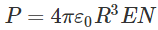From the above expression it is found that the electronic polarization or atomic polarization is dependent upon the radius (or volume) of the atom and the number of atoms presents in unit volume of the material.

Want To Learn Faster? 🎓
Get electrical articles delivered to your inbox every week.
No credit card required—it’s 100% free.